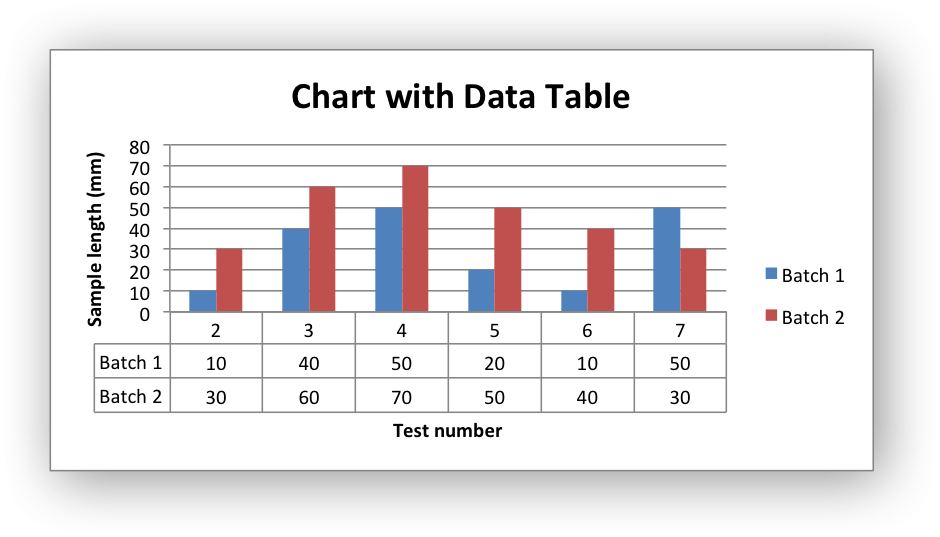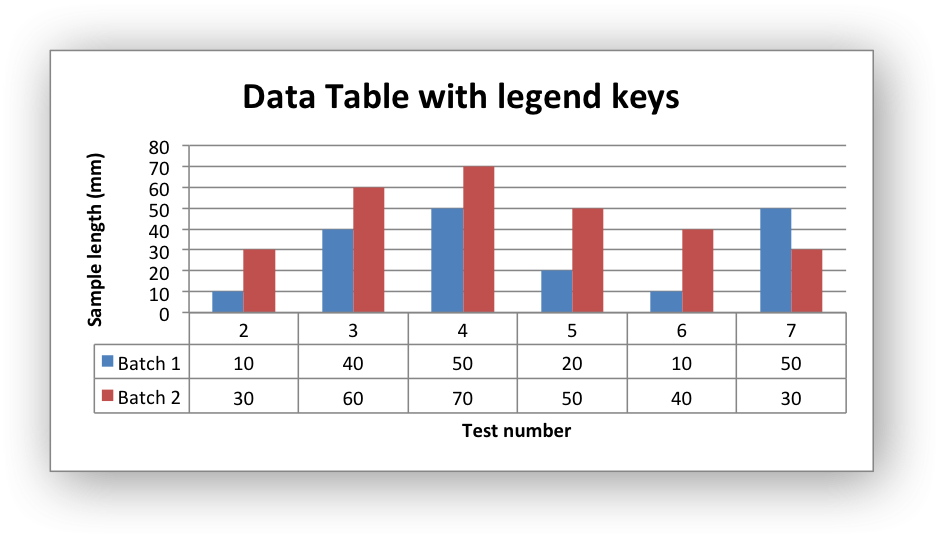# Example: Charts with Data Tables

Example of creating charts with data tables.

Chart 1 in the following example is a column chart with default data table:Chart 2 is a column chart with default data table with legend keys:```#######################################################################
#
# An example of creating Excel Column charts with data tables using
# Python and XlsxWriter.
#
# Copyright 2013-2023, John McNamara, jmcnamara@cpan.org
#
import xlsxwriter

workbook = xlsxwriter.Workbook("chart_data_table.xlsx")

# Add the worksheet data that the charts will refer to.
headings = ["Number", "Batch 1", "Batch 2"]
data = [
[2, 3, 4, 5, 6, 7],
[10, 40, 50, 20, 10, 50],
[30, 60, 70, 50, 40, 30],
]

worksheet.write_column("A2", data)
worksheet.write_column("B2", data)
worksheet.write_column("C2", data)

#######################################################################
#
# Create a column chart with a data table.
#

# Configure the first series.
{
"name": "=Sheet1!\$B\$1",
"categories": "=Sheet1!\$A\$2:\$A\$7",
"values": "=Sheet1!\$B\$2:\$B\$7",
}
)

# Configure second series. Note use of alternative syntax to define ranges.
{
"name": ["Sheet1", 0, 2],
"categories": ["Sheet1", 1, 0, 6, 0],
"values": ["Sheet1", 1, 2, 6, 2],
}
)

# Add a chart title and some axis labels.
chart1.set_title({"name": "Chart with Data Table"})
chart1.set_x_axis({"name": "Test number"})
chart1.set_y_axis({"name": "Sample length (mm)"})

# Set a default data table on the X-Axis.
chart1.set_table()

# Insert the chart into the worksheet (with an offset).
worksheet.insert_chart("D2", chart1, {"x_offset": 25, "y_offset": 10})

#######################################################################
#
# Create a column chart with a data table and legend keys.
#

# Configure the first series.
{
"name": "=Sheet1!\$B\$1",
"categories": "=Sheet1!\$A\$2:\$A\$7",
"values": "=Sheet1!\$B\$2:\$B\$7",
}
)

# Configure second series.
{
"name": "=Sheet1!\$C\$1",
"categories": "=Sheet1!\$A\$2:\$A\$7",
"values": "=Sheet1!\$C\$2:\$C\$7",
}
)

# Add a chart title and some axis labels.
chart2.set_title({"name": "Data Table with legend keys"})
chart2.set_x_axis({"name": "Test number"})
chart2.set_y_axis({"name": "Sample length (mm)"})

# Set a data table on the X-Axis with the legend keys shown.
chart2.set_table({"show_keys": True})

# Hide the chart legend since the keys are shown on the data table.
chart2.set_legend({"position": "none"})

# Insert the chart into the worksheet (with an offset).
worksheet.insert_chart("D18", chart2, {"x_offset": 25, "y_offset": 10})

workbook.close()
```